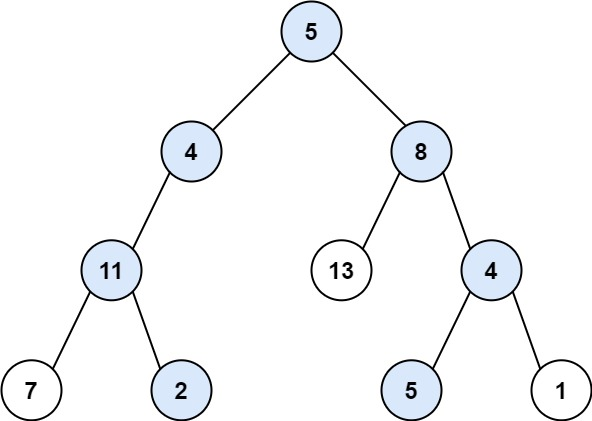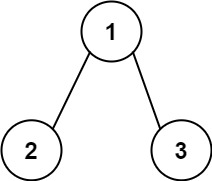GeetCode Hub

Given the root of a binary tree and an integer targetSum, return all root-to-leaf paths where each path's sum equals targetSum.

A leaf is a node with no children.

Example 1:Input: root = [5,4,8,11,null,13,4,7,2,null,null,5,1], targetSum = 22
Output: [[5,4,11,2],[5,8,4,5]]

Example 2:Input: root = [1,2,3], targetSum = 5
Output: []

Example 3:

Input: root = [1,2], targetSum = 0
Output: []

Constraints:

• The number of nodes in the tree is in the range [0, 5000].
• -1000 <= Node.val <= 1000
• -1000 <= targetSum <= 1000

/** * Definition for a binary tree node. * public class TreeNode { * int val; * TreeNode left; * TreeNode right; * TreeNode() {} * TreeNode(int val) { this.val = val; } * TreeNode(int val, TreeNode left, TreeNode right) { * this.val = val; * this.left = left; * this.right = right; * } * } */ class Solution { public List<List<Integer>> pathSum(TreeNode root, int targetSum) { } }